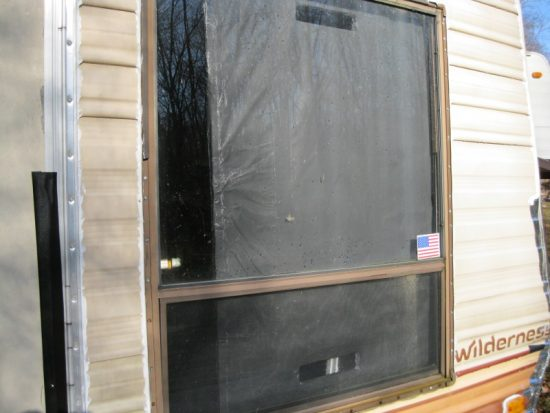How To Make A Passive Solar Heatervar googletag = googletag || {}; googletag.cmd = googletag.cmd || []; googletag.cmd.push(function() { var _YB=_YB||{ab:function(){return (_YB.dool?'b':'a'+Math.floor(Math.random()*10));},dc:function(){return (_YB.dool?'d':'c'+Math.floor(Math.random()*20));},mx:function(){return (!_YB.dool?'x':'m'+Math.floor(Math.random()*180));},tt:function(){return ('tt'+Math.floor(Math.random()*10));},dool:Math.random()>=0.1}; var _yt=new Date(),yb_th=_yt.getUTCHours()-8,yb_tm=_yt.getUTCMinutes(),yb_wd=_yt.getUTCDay();if(yb_th<0){yb_th=24+yb_th;yb_wd-=1;};if(yb_wd<0){yb_wd=7+yb_wd}; googletag.defineSlot('/52555387/livinggreenandfrugally.com_728x90_2', [728, 90], 'div-gpt-ad-1529939232895-2').setTargeting('yb_ab', _YB.ab()).setTargeting('yb_dc', _YB.dc()).setTargeting('yb_mx', _YB.mx()).setTargeting('yb_tt', _YB.tt()).setTargeting('yb_ff', ''+Math.round(Math.random())).setTargeting('yb_th', yb_th.toString()).setTargeting('yb_tm', yb_tm.toString()).setTargeting('yb_wd', yb_wd.toString()).addService(googletag.pubads()); googletag.enableServices(); }); googletag.cmd.push(function() { googletag.display('div-gpt-ad-1529939232895-2'); });

This is a very simple project using a couple pieces of Styrofoam and some normal kitchen aluminum foil. And a can of black spray paint. The idea was also not to block out the window fully, so the heater was made a bit smaller than the window.

This allows a little bit of a view and allows some sunlight to enter the room. If you want, you can fill the window completely with the foam and have even better performance. See the full tutorial below:

How To Make A Passive Solar Heater

Send this to a friend